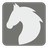The Fastest Horse
Elementary+
English RU

We have some horse racing statistics (each horse’s time in each race)
You have to find the number of the horse which has the most wins.
For example: if the results are [[“1:13”, “1:26”, “1:11”], [“1:10”, “1:18”, “1:14”], [“1:20”, “1:23”, “1:15”]] then the 3rd horse is the fastest, because it has won 2 races out of 3.
Every element in the list - is a string in format m:ss, for example, "1:05" or "2:22". 1:00 <= time <= 5:00Input: Racing time as an array of arrays.

Output: The number of the fastest horse that has the most wins (Important: in this task the horse numbers starts from "1", not from "0")

Example:

```fastest_horse([[“1:13”, “1:26”, “1:11”], [“1:10”, “1:18”, “1:14”], [“1:20”, “1:23”, “1:15”]]) == 3
```

How it is used: For data analysis and statistics (finding the needed elements in the huge amount of data).

Precondition:
2 <= horses <= 10
1 <= races <= 10
1:00 <= time <= 5:00
There is only 1 fastest horse in each test